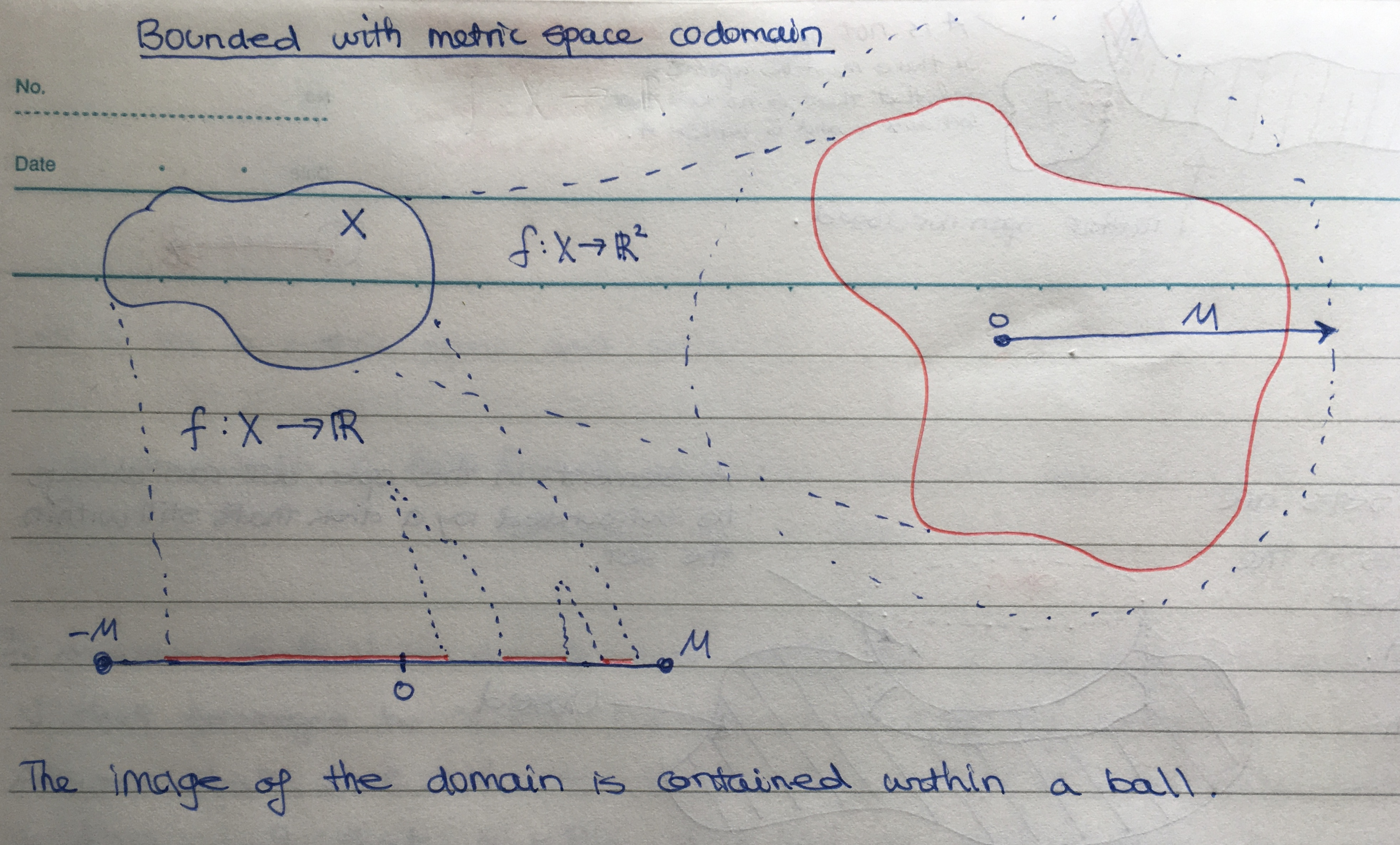Math and science::Topology

# Compactness. Motivation

What would we have to assume about a topological space $$X$$ in order to prove that every continuous map $$f : X \to \mathbb{R}$$ is bounded?

### Reasoning summary

Condensed version.

• What is the definition of being bounded?
• What are some cases where we know functions are bounded?
• The most basic case: the domain is [...].
• Can we generalize this?
• A little more general:
For a given $$f$$, the domain can be covered by a [...], each of which has an image that is bounded.
• This is restricted to a given $$f$$. Can we generalize?
• Every continuous $$f$$ induces a neighbourhood around each $$x \in X$$, and $$f$$ will be bounded for each of these neigbourhoods. (Remember, the codomain is $$\mathbb{R}$$).
• So, by the definition of continuity, we have a cover where each subset is bounded.
• Sadly, this set of neighbourhoods could be [...].
• Thus we arrive at our requirement: every open cover must have [...].
• Which means, every continuous $$f$$ will induce an arbitrary open cover on $$X$$ (by [definition of what?]), and we impose that this cover has a finite subcover.